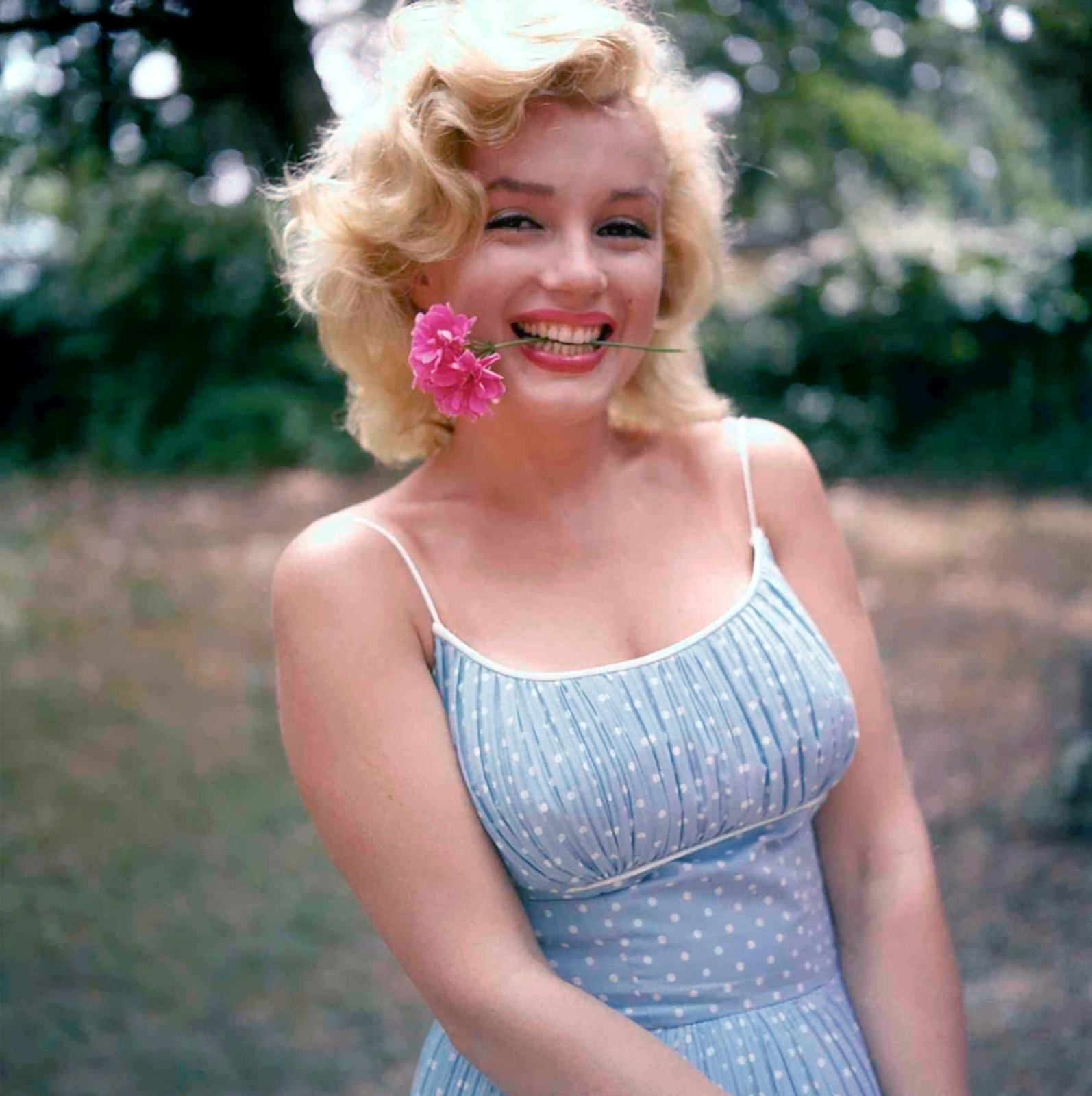Shen# 主题介绍

Oct 31, 2015 • 2 min read

• 封面图片。通过设置 cover-image 来添加，图片的宽度尽量超过 800px，通过自动缩小来适应页面。页面中的图片左对齐，没有居中处理。如果需要居中的话，可以在 css 文件 img 中设置 margin: 0 auto;
• 一级标题。没有采用 # H1 # 来添加，而是通过 title 来设置博客标题。
• 二级标题。直接采用 Markdown 语言 ## H2 ## 来添加，在二级标题上有一道灰线，作为分隔线。
• 三级及以下标题。方法同二级标题 ### H3 ### ，但是没有分隔线。
• 段落。 行高设置为 1.8em，段前段后空 1em。
• 引用。在段前采用 >，引用文字为灰色，呈斜体。
• 图片。不建议采用 ![figure alt](figure url)，因为这种类型的图片已经设置了一种特殊的 css 样式。而建议采用 html 的语法 <div class="figure">...</div>
• 高亮代码。在代码前后分别添加 {\% highlight css \%}{\% endhighlight \%}
• 公式。采用 <div class="formula">...</div>
• 视频。采用 <div class="video">...</div>

## 二级标题

### 三级标题

• item 1：橄榄树
• item 2：月琴
• item 3：美丽岛

#### 图片一张风景图片

#### 公式

\begin{eqnarray} \dot{x}& =& \sigma(y-x) \\ \dot{y}& =& \rho x - y - xz \\ \dot{z}& = &-\beta z + xy \end{eqnarray} \begin{eqnarray} \frac{\mathrm{d}D_e}{\mathrm{d}N} & = &[1-(1-D)^{\beta+1}]^{\alpha(\sigma_{max}\bar{\sigma})} \nonumber\\ & & \Big[\frac{\sigma_{max}-\bar{\sigma}}{M_0 (1-b_2\bar{\sigma})(1-D)}\Big]^{\beta} \end{eqnarray} \begin{equation*} E = mc^2 \end{equation*}

#### 表格

Left-Aligned Center Aligned Right Aligned
col 3 is some wordy text $1600 col 2 is centered$12
zebra stripes are neat \$1

GitHub